Home > A2C > Chapter 8 > Lesson 8.2.4 > Problem8-158

8-158.
1. Susan knew how to shift y = sin x to get the graph below, but she wondered if it would be possible to get the same graph by shifting y = cos x. Homework Help ✎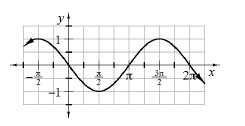1. Is it possible to write a cosine function for this graph?

2. If you think it is possible, find an equation that does it. If you think it is impossible, explain why.

3. Adlai said, “I can get that graph without shifting to the right or left.” What equation did he write?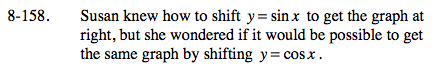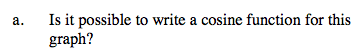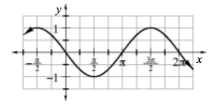Yes, it is.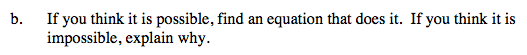The graph of y = cos x starts at a maximum. This graph has a maximum at

$x = - \frac{\pi}{2} .$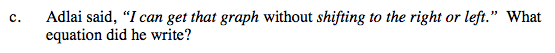y = −sin(x)